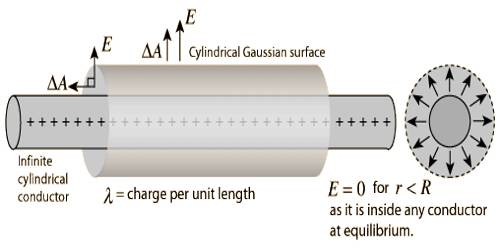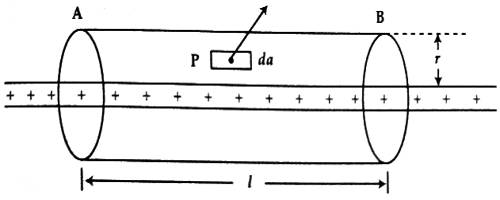# Gauss’s Law to determine Electric Field due to Charged Line

Gauss’s Law to determine Electric field due to charged line of infinite length

The electric field of an infinite line charge with a uniform linear charge density can be obtained by a using Gauss’ law. Let us consider a uniformly charged wire charged line of infinite length whose charge density or charge per unit length is λ. Let a point P at a distance r from the charge line be considered [Figure]. Electric field E at point P is to be determined.Let us consider a Gaussian cylinder of length ‘l’ and radius ‘r’ taking the charge line as axis. Then the point P will be on the curved surface of the cylinder. The magnitude of the electric field E of all points on the curved surface is same and direction is outward along normal to the curved surface. So, at point P electric field E and field vector da are in the same direction. Hence according to Gauss’s law,

ε0 § E.d a = ε0 §Eda = λl.

or, ε0E§ Eda = λl.

or, ε0E (2πrl)a = λl

So, E = λ/ (2πε0r)

E acts perpendicular to the normal of the two circular surfaces of Gaussian cylinder. Hence, for both the curved surfaces ε0§E.d a = 0. So, the required electric field, E = λ / (2πε0r).